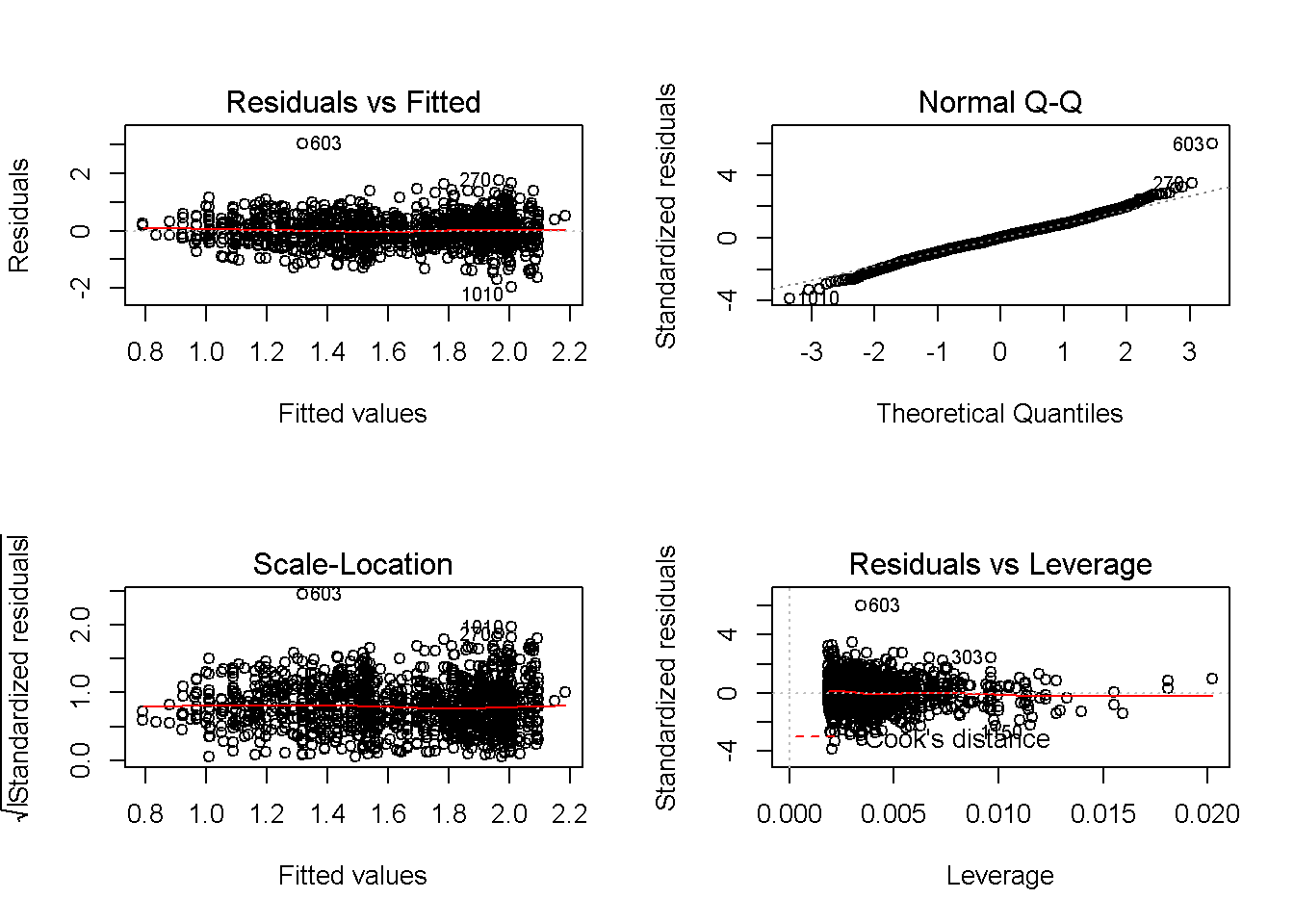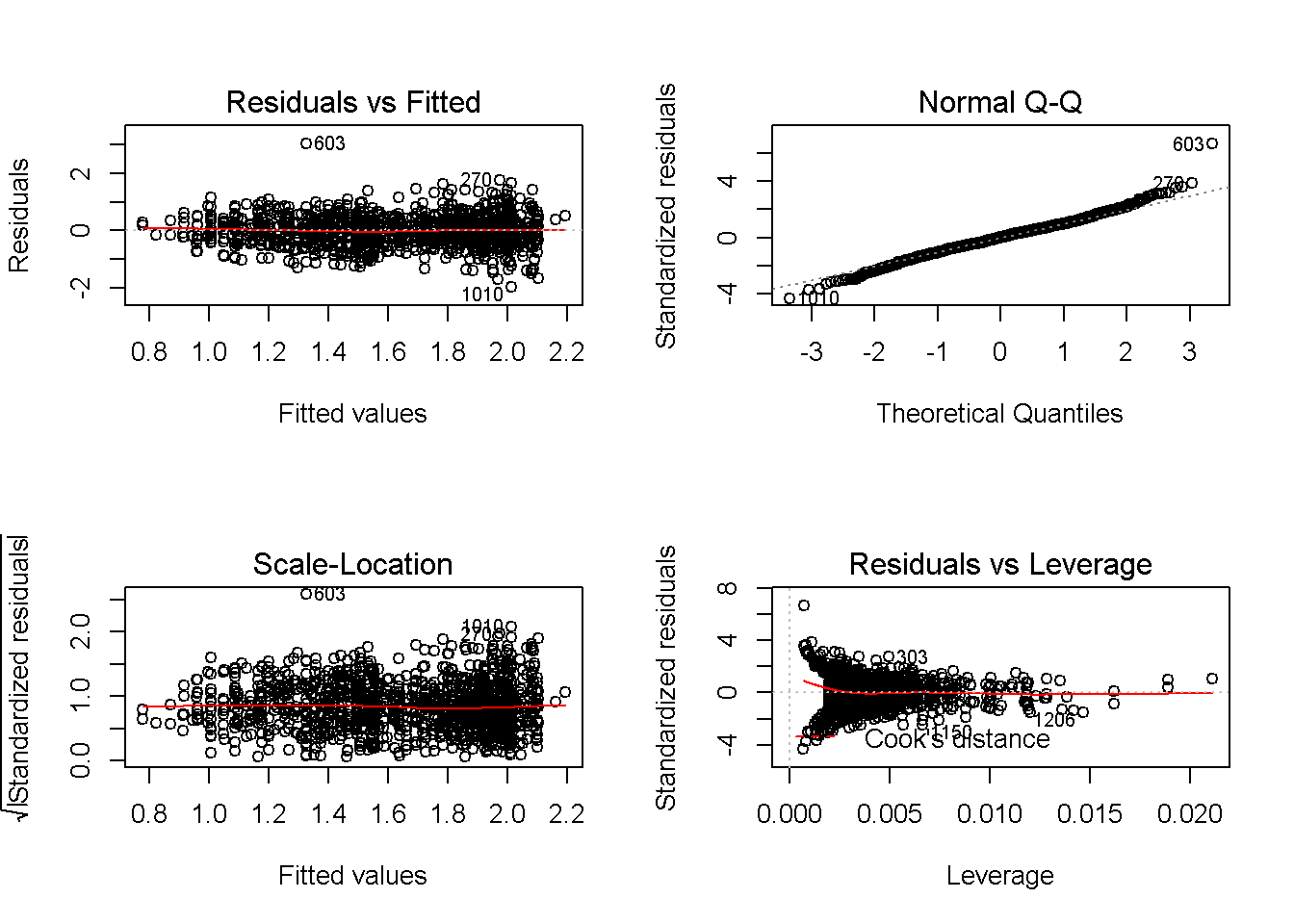ECON2206 Assignment2 2018 2nd Sem
(Problem1) * Originally, STATA assignment * Adpated to R

The file beauty.dta collects information on wages hourly wage, years of experience and gender for 1; 260 individuals. We also have information on a subjective index of beauty (looks) measured from 1 to 5.

$lwage = \beta_0 + exper + exper^2 + \beta_3 looks + \beta_4female + u \ (1)$

1. Required packages
library(tidyverse) #data wrangling
library(modelr)    #modelling
library(car)       #linearHypothesis()
library(MASS)      #robust regression
1. Estimate the parameter of the econometric model in equation (1). What is the estimated effect of experience on wages?

Importing STATA data file(.dta) into R
1) open STATA
2) open the corresponding data file(.dta)
3) type “saveold (file_name).dta, version(12)”
4) import the saved file into R using read.dta function

beauty <- read.dta("C:/Users/Kang/Desktop/UNSW/S2/ECON2206/STATA and Assignments/beauty(1).dta")
colnames(beauty)
##   "wage"     "lwage"    "belavg"   "abvavg"   "exper"    "looks"
##   "union"    "goodhlth" "black"    "female"   "married"  "south"
##  "bigcity"  "smllcity" "service"  "expersq"  "educ"
beauty %>% head()
##    wage    lwage belavg abvavg exper looks union goodhlth black female
## 1  5.73 1.745715      0      1    30     4     0        1     0      1
## 2  4.28 1.453953      0      0    28     3     0        1     0      1
## 3  7.96 2.074429      0      1    35     4     0        1     0      1
## 4 11.57 2.448416      0      0    38     3     0        1     0      0
## 5 11.42 2.435366      0      0    27     3     0        1     0      0
## 6  3.91 1.363537      0      0    20     3     0        0     0      1
##   married south bigcity smllcity service expersq educ
## 1       1     0       0        1       1     900   14
## 2       1     1       0        1       0     784   12
## 3       0     0       0        1       0    1225   10
## 4       1     0       1        0       1    1444   16
## 5       1     0       0        1       0     729   16
## 6       1     0       1        0       0     400   12

A. estimated parameters

mod1 <- lm(lwage ~ exper + expersq + looks + female, data = beauty)
mod1
##
## Call:
## lm(formula = lwage ~ exper + expersq + looks + female, data = beauty)
##
## Coefficients:
## (Intercept)        exper      expersq        looks       female
##   1.0783855    0.0462051   -0.0008054    0.0887511   -0.4664837

B. estimated effect of experience on avg

impact_exper <- function(current_exper) {
coef(mod1) + 2*coef(mod1) * current_exper #coef(mod1): exper regressor
}                                                 #coef(mod1): expersq regressor

impact_exper(5) #ex) estimated effect on log(wage) of one more yr of exper for a person with 5 yrs of exper
##      exper
## 0.03815152
1. What can we say about the effect of an increase of one point in the looks scale on wages?
coef(mod1)
##      looks
## 0.08875113
1. Is there any evidence of functional form misspecification for equation (1)?

\text{RESET test} \\ \begin{align} &lwage = \gamma_1exper+\gamma_2exper^2 + \gamma_3looks + \gamma_4female + \gamma_5fitted^2 + \gamma_6fitted^3 \ \text{(unrestricted model)} \\ &lwage = \beta_0 + exper + exper^2 + \beta_3 looks + \beta_4female + u \ (1) \ \text{(restricted model)} \\ \end{align} \\ \begin{align} &H_0: \gamma_5 = \gamma_6 = 0 \ &\text{(no misspecification)}\\ &H_1: \text{~} H_0 \ &\text{(misspecifcation)} \end{align}

1) create fitted^2, fitted^3 values (column)

beauty$fitted1 <- predict(mod1) beauty$fitted2 <- predict(mod1)^2
beautyfitted3 <- predict(mod1)^3 2) run the unrestricted model mod1_1 <- lm(lwage ~ exper + expersq + looks + female + fitted2 + fitted3, data = beauty) 3) joint F-test linearHypothesis(mod1_1, c("fitted2=0", "fitted3=0")) #H0: cannot be rejected ## Linear hypothesis test ## ## Hypothesis: ## fitted2 = 0 ## fitted3 = 0 ## ## Model 1: restricted model ## Model 2: lwage ~ exper + expersq + looks + female + fitted2 + fitted3 ## ## Res.Df RSS Df Sum of Sq F Pr(>F) ## 1 1255 321.21 ## 2 1253 320.39 2 0.81863 1.6008 0.2022  #No significant misspecification 1. How would you test the hypothesis that beauty has the same effect on wages both for males and females? Provide a p-value for such test. 1) create an interaction term * between female and looks * if this term is significant, different effects of looks on each different gender confirmed beauty <- beauty %>% mutate(femlooks = female*looks) 2) model estimates + hypothesis testing mod3 <- lm(lwage ~ exper + expersq + looks + female + femlooks, data = beauty) summary(mod3)  ## ## Call: ## lm(formula = lwage ~ exper + expersq + looks + female + femlooks, ## data = beauty) ## ## Residuals: ## Min 1Q Median 3Q Max ## -1.9626 -0.3068 0.0111 0.3068 3.0112 ## ## Coefficients: ## Estimate Std. Error t value Pr(>|t|) ## (Intercept) 1.1347492 0.0977813 11.605 < 2e-16 *** ## exper 0.0466609 0.0046682 9.995 < 2e-16 *** ## expersq -0.0008166 0.0001039 -7.862 8.12e-15 *** ## looks 0.0700875 0.0267244 2.623 0.00883 ** ## female -0.6228310 0.1410797 -4.415 1.10e-05 *** ## femlooks 0.0489631 0.0431046 1.136 0.25621 ## --- ## Signif. codes: 0 '***' 0.001 '**' 0.01 '*' 0.05 '.' 0.1 ' ' 1 ## ## Residual standard error: 0.5059 on 1254 degrees of freedom ## Multiple R-squared: 0.2789, Adjusted R-squared: 0.276 ## F-statistic: 96.99 on 5 and 1254 DF, p-value: < 2.2e-16 #not significant (p-value: 25.6%) 1. Is there any evidence of heteroskedasticity in equation (1)? Provide an heteroskedas- ticity robust estimate of the parameters in equation (1). A. BP test [ \begin{align} \text{Theoratical background}\\ &Var(u|X) = E(u^2|X) - [E(u|X)]^2 = E(u^2|X) \ \text{(zero conditional mean)} \\ \text{In case of homoskedasticity,} \\ &Var(u|X) = Var(u) = \sigma^2 \\ \text{and accordingly} \\ &E(u^2|X) = E(u^2) = \sigma^2 \\ \text{Therefore,} \\ &H_0: u^2\ \text{has no linear relationship with respect to x (homoskedasticity)} \\ &H_1: \text{~} H_0 \end{align} ] beautyresid <- resid(mod1)
beauty\$resid2 <- resid(mod1)^2

hetero1 <- lm(resid2 ~ exper + expersq + looks + female, data = beauty)
summary(hetero1)
##
## Call:
## lm(formula = resid2 ~ exper + expersq + looks + female, data = beauty)
##
## Residuals:
##     Min      1Q  Median      3Q     Max
## -0.3082 -0.2241 -0.1507  0.0353  8.9580
##
## Coefficients:
##               Estimate Std. Error t value Pr(>|t|)
## (Intercept)  1.218e-01  7.911e-02   1.539  0.12399
## exper        1.133e-02  4.367e-03   2.594  0.00961 **
## expersq     -2.250e-04  9.709e-05  -2.317  0.02064 *
## looks        8.844e-03  1.979e-02   0.447  0.65505
## female       1.595e-02  2.907e-02   0.549  0.58338
## ---
## Signif. codes:  0 '***' 0.001 '**' 0.01 '*' 0.05 '.' 0.1 ' ' 1
##
## Residual standard error: 0.475 on 1255 degrees of freedom
## Multiple R-squared:  0.005741,   Adjusted R-squared:  0.002572
## F-statistic: 1.812 on 4 and 1255 DF,  p-value: 0.1242
#no heteroskedasticity by BP test (F-statistic)

B. White test

$u^2 = \delta_0 + \delta_1\hat{y} + \delta_2\hat{y}^2 + \epsilon \\ H_0: \delta_1 = \delta_2 =0$

hetero2 <- lm(resid2 ~ fitted1 + fitted2, data = beauty)
summary(hetero2) 
##
## Call:
## lm(formula = resid2 ~ fitted1 + fitted2, data = beauty)
##
## Residuals:
##     Min      1Q  Median      3Q     Max
## -0.2994 -0.2261 -0.1586  0.0351  8.9843
##
## Coefficients:
##             Estimate Std. Error t value Pr(>|t|)
## (Intercept)   0.4714     0.3467   1.360    0.174
## fitted1      -0.3549     0.4521  -0.785    0.433
## fitted2       0.1306     0.1430   0.913    0.361
##
## Residual standard error: 0.4755 on 1257 degrees of freedom
## Multiple R-squared:  0.002035,   Adjusted R-squared:  0.000447
## F-statistic: 1.282 on 2 and 1257 DF,  p-value: 0.278
#no heteroskedasticity by White test (F-statistic)

C. Robust Regression

mod4 <- rlm(lwage ~ exper + expersq + looks + female, data = beauty)
1. Bonus: Residual Analysis
par(mfrow = c(2,2))
plot(mod1) #No significant problem observedpar(mfrow = c(2,2))
plot(mod4) #robust model